PHY294H - Lecture 26

A time varying magnetic field

So far we have considered cases where the applied magnetic field is constant and uniform while the area through which the field passes has been altered by mechanical means. Consider now a region of space in which the magnetic field is increasing as B(t) = B0 + B1t. we want to find the electric field that this changing magnetic field induces. We use Faraday's law to find,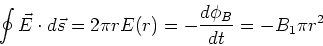(1)

Solving gives,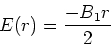(2)

Recall that if we have a time varying electric field E(t) = E0 + E1 t, then we carry out an analogous calculation using the Maxwell displacement term to find,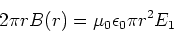(3)

which gives,(4)

Example - A rotating rod

Consider a conducting rod at angle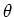to the x-axis. The rod is rotated at constant angular speed w so the angle increases as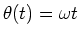. Close the loop by making a circular pie segment with the x-axis and the rod as its bounding radii. The rate of change of flux is given by,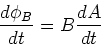(5)

The area of the segment is given by,(6)

We then have,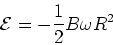(7)

where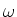is the angular speed of the rod.

Example: Moving crossbar

Consider a rectangular loop where one of the sides is a freely movable crossbar, while the other sides are fixed. The loop lies in constant B field which is parallel with the loop normal. If the wire making up the loop and the crossbar has resistance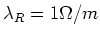, the crossbar has length L=10cm, the crossbar is moving at speed 5m/sand the magnetic field has magnitude B = 1T, find: a) The force required to maintain the speed of the crossbar; b) The power dissipated in the rectangular loop.

The induced emf is given by,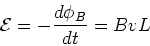(8)

The resistance of the loop is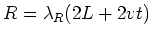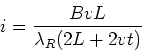(9)

The force on the crossbar is,

 F = i L B (10)

in a direction opposite to the velocity of the crossbar. The power (work per unit time) of the external force is,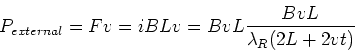(11)

The instantaneous electrical power dissipated is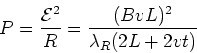(12)

The power dissipated in the circuit is thus provided by the external force which is doing work to move the crossbar. Note that if the resistance of the loop is very large, the work is small. If the loop in insulating, there is an induced emf but no current flow and hence no dissipation. In that case no force is required to move the loop out of the magnetic field.

Example - Eddy currents

A piece of metal dropped through a magnetic field also has induced electric fields and induced currents. These currents are called eddy currents and can be quite large. They lead to resistive losses, ie.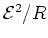losses. It is evident that the larger the resistance the smaller the losses, for fixed induced emf. One strategy for reducing eddy current losses is then to laminate or in other ways increase the resistance of a piece of metal.

A bar magnet and a loop

If we move a bar magnet toward a conducting loop, there is an induced current in the loop. The induced current produces a magnetic field that looks like an induced bar magnet. The magnitude of the induced bar magnet is dependent on the resistance of the loop, the size of the real bar magnet and the speed at which we move the real bar magnet toward the loop.

An important special case - A superconductor

Superconductors are a special case where the induced currents occur even in a constant magnetic field. That is, even constant fields induce currents which oppose the applied field. This effect leads to a variety of new applications such as magnetic levitation.